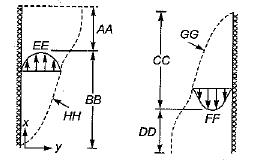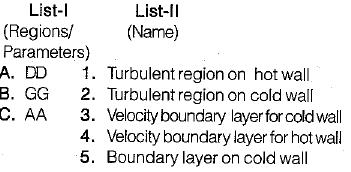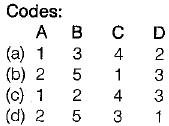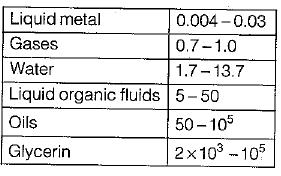Courses

# Test: Heat Transfer By Natural Convection - 1

## 10 Questions MCQ Test Topicwise Question Bank for Mechanical Engineering | Test: Heat Transfer By Natural Convection - 1

Description
This mock test of Test: Heat Transfer By Natural Convection - 1 for Chemical Engineering helps you for every Chemical Engineering entrance exam. This contains 10 Multiple Choice Questions for Chemical Engineering Test: Heat Transfer By Natural Convection - 1 (mcq) to study with solutions a complete question bank. The solved questions answers in this Test: Heat Transfer By Natural Convection - 1 quiz give you a good mix of easy questions and tough questions. Chemical Engineering students definitely take this Test: Heat Transfer By Natural Convection - 1 exercise for a better result in the exam. You can find other Test: Heat Transfer By Natural Convection - 1 extra questions, long questions & short questions for Chemical Engineering on EduRev as well by searching above.
QUESTION: 1

Solution:
QUESTION: 2

Solution:
QUESTION: 3

### Choose the wrong statement with respect to Nusselt number and convective heat transfer coefficient

Solution:
QUESTION: 4

Which one of the following number represents the ratio of kinematic viscosity to thermal diffusivity?

Solution:

Prandtl number is the ratio of two transport properties i.e the ratio of momentum diffusivity and thermal diffusivity. Prandtl number controls the relation between the velocity and temperature distributions.

QUESTION: 5

Consider the following boundary layer for natural convection on a vertical plate and match accordingly:Solution:

AA : Turbulent region on hot wall
BB : Laminar region on hot wall
CC: Laminar region on cold wall
DD: Turbulent region on cold wall
EE : Velocity profile for hot wall
FF : Velocity profile for cold wall
GG: Boundary layer for cold wall
HH : Boundary layer for hot wall..

QUESTION: 6

Which of the following parameter is a measure of the relative importance of natural convection in relation to forced convection?
All empirical parameters have their usual meaning.

Solution:

If (Gr/Re2) << 1, flow is primarily by forced convection
If (Gr/Re2) >> 1, flow is dominant by natural convection
If (Gr/Re2) ≌ 1, natural and forced convection are of same magnitude.

QUESTION: 7

This value governs the transition from laminar to turbulent flow in natural convection:

Solution:

The Grashoff number in natural convection plays the same role as the Reynolds number in forced convection because it is the ratio of buoyancy force to the viscous force.

QUESTION: 8

The empirical correlation for natural convection is represented by
All empirical parameters have their usual meaning.

Solution:
QUESTION: 9

The Prandtl number will be lowest for

Solution:QUESTION: 10

In respect of free convection over a vertical flat plate, the Nusselt number for laminar and turbulent flow varies respectively as

Solution:

for vertical plate,
Nu = 0.59 Ra1/4 for 104 < Ra < 109
Nu = 0.13 Ra1/3 for 1010 < Ra < 1013